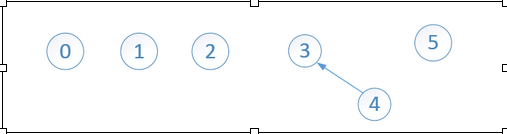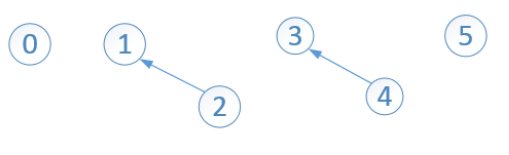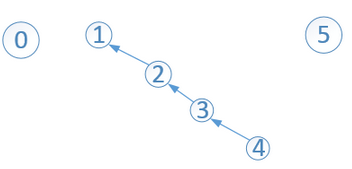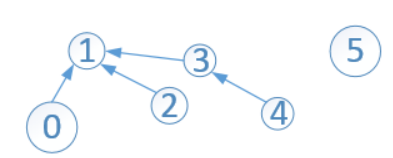## 二、并查集的存储结构及实现分析

①存储结构

``````public class DisjSets {
private int[] s;
private int count;//记录并查集中子集合的个数(子树的个数)

public DisjSets(int numElements) {
//构造函数，负责初始化并查集
}

public void unionByHeight(int root1, int root2){
//union操作
}

public int find(int x){
//find 操作
}
}
````````````public DisjSets(int numElements) {
s = new int[numElements];
count = numElements;
//初始化并查集,相当于新建了s.length 个互不相交的集合
for(int i = 0; i < s.length; i++)
s[i] = -1;//s[i]存储的是高度(秩)信息
}
``````

②基本操作实现

Union操作就是将两个不相交的子集合合并成一个大集合。简单的Union操作是非常容易实现的，因为只需要把一棵子树的根结点指向另一棵子树即可完成合并。``````public void union(int root1, int root2){
s[root2] = root1;//将root1作为root2的新树根
}
``````

Find操作就是查找某个元素所在的集合，返回该集合的代表元素。在union(3,4) 和 union(1,2)后，并查集如下：find(2)返回1，find(0)返回0。因为 结点3 和 结点4 在同一个集合内，find(4)返回3，find(3)返回3。

`````` public int find(int x){
if(s[x] < 0)
return x;
else
return find(s[x]);
}
``````

（数组中索引 i 处的元素 s[i] 小于0，表示 结点i 是根结点.....）

## 三、Union/Find的改进----Quick Union/Find``````/**
*
* @param root1 并查集中以root1为代表的某个子集
* @param roo2 并查集中以root2为代表的某个子集
* 按高度(秩)合并以root1 和 root2为代表的两个集合
*/
public void unionByHeight(int root1, int root2){
if(find(root1) == find(root2))
return;//root1 与 root2已经连通了

if(s[root2] < s[root1])//root2 is deeper
s[root1] = root2;
else{
if(s[root1] == s[root2])//root1 and root2 is the same deeper
s[root1]--;//将root1的高度加1
s[root2] = root1;//将root2的根(指向)更新为root1
}

count--;//每union一次,子树数目减1
}
``````

#### 使用了路径压缩的Find的操作

``````public int find(int x){
if(s[x] < 0)//s[x]为负数时,说明 x 为该子集合的代表(也即树根), 且s[x]的值表示树的高度
return x;
else
return s[x] = find(s[x]);//使用了路径压缩,让查找路径上的所有顶点都指向了树根(代表节点)
//return find(s[x]); 没有使用 路径压缩
}
``````

#### 关于路径压缩对按”秩“求并的兼容性问题-3表示的高度为2，但是树的高度实际上已经变成了1（关于路径压缩对按秩合并有影响，我一直有个疑问，希望有大神指点啊）。。。。

## 五、完整代码

``````
public class DisjSets {
private int[] s;
private int count;//记录并查集中子集合的个数(子树的个数)

public DisjSets(int numElements) {
s = new int[numElements];
count = numElements;
//初始化并查集,相当于新建了s.length 个互不相交的集合
for(int i = 0; i < s.length; i++)
s[i] = -1;//s[i]存储的是高度(秩)信息
}

/**
*
* @param root1 并查集中以root1为代表的某个子集
* @param roo2 并查集中以root2为代表的某个子集
* 按高度(秩)合并以root1 和 root2为代表的两个集合
*/
public void unionByHeight(int root1, int root2){
if(find(root1) == find(root2))
return;//root1 与 root2已经连通了

if(s[root2] < s[root1])//root2 is deeper
s[root1] = root2;
else{
if(s[root1] == s[root2])//root1 and root2 is the same deeper
s[root1]--;//将root1的高度加1
s[root2] = root1;//将root2的根(指向)更新为root1
}

count--;//每union一次,子树数目减1
}

public void union(int root1, int root2){
s[root2] = root1;//将root1作为root2的新树根
}

public void unionBySize(int root1, int root2){

if(find(root1) == find(root2))
return;//root1 与 root2已经连通了

if(s[root2] < s[root1])//root2 is deeper
s[root1] = root2;
else{
if(s[root1] == s[root2])//root1 and root2 is the same deeper
s[root1]--;//将root1的高度加1
s[root2] = root1;//将root2的根(指向)更新为root1
}

count--;//每union一次,子树数目减1
}

public int find(int x){
if(s[x] < 0)//s[x]为负数时,说明 x 为该子集合的代表(也即树根), 且s[x]的值表示树的高度
return x;
else
return s[x] = find(s[x]);//使用了路径压缩,让查找路径上的所有顶点都指向了树根(代表节点)
//return find(s[x]); 没有使用 路径压缩
}

public int find0(int x){
if(s[x] < 0)
return x;
else
return find0(s[x]);
}

public int size(){
return count;
}
}
``````